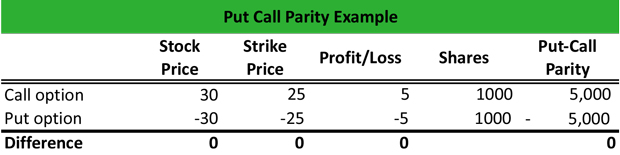# What is a Put-Call Parity?

Definition: Under the put-call parity, a long European call and a short European put with an identical strike price, underlying asset, and maturity, should also have an identical return.

## What Does Put-Call Parity Mean?

What is the definition of put call parity mean? The put-call parity holds that the relationship between a call and a put with the same underlying asset, strike price, and maturity should not yield any profit of loss. This happens because the call option expects that the price will rise further and the put option expects that the price will decline further.

Because the risk and return are identical, the return is the same, yielding no profit or loss. In the case, that the call or the put option diverge from the call-put parity, then there is an arbitrage opportunity.

Let’s look at an example.

## Example

The put-call parity formula holds that the difference between the price of the call option today and the put option today is equal to the stock price today minus the strike price discounted by the risk-free rate and the time remaining until maturity.

Hence:

C – P = S – K / ( 1 + r)T

Mark purchases a European call option for a stock that trades at \$30. The strike price is \$25, the maturity is in 6 months, and Mark pays \$5 to acquire the call option. If at maturity the stock trades at \$40, Mark will exercise his call option and realize a profit of \$40 – \$30 = \$10- \$5 = \$5.

Mark writes also a European put option for the same stock, with a strike price of \$25, maturity in 6 months, and a cost of \$5 to sell the put. If at maturity the stock trades at \$20, the option holder will sell Mark the stock at \$30, and Mark will get \$30 – \$25 = \$5. So, he will break-even.## Summary Definition

Define Put Call Parity: Put-Call Parity means investment nuance where a put and call are placed on the same asset with the same strike price and have the same results.